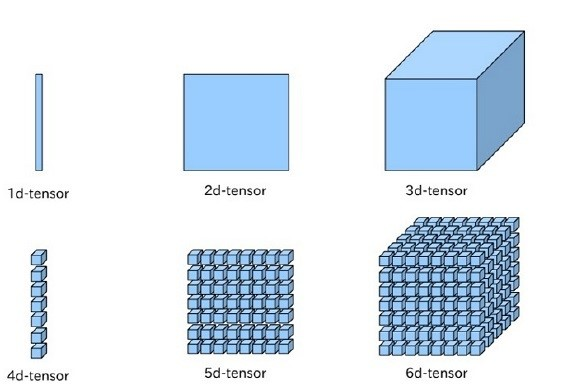# Fluid编程指南¶

• Fluid有哪些核心概念
• 如何在fluid中定义运算过程
• 如何使用executor运行fluid操作
• 如何从逻辑层对实际问题建模
• 如何调用API（层，数据集，损失函数，优化方法等等）

## 使用Tensor表示数据¶

Fluid和其他主流框架一样，使用Tensor数据结构来承载数据。1. 模型中的可学习参数

```w = fluid.layers.create_parameter(name="w",shape=,dtype='float32')
```

```import paddle.fluid as fluid
y = fluid.layers.fc(input=x, size=128, bias_attr=True)
```

2. 输入输出Tensor

Fluid 中使用 `fluid.layers.data` 来接收输入数据， `fluid.layers.data` 需要提供输入 Tensor 的形状信息，当遇到无法确定的维度时，相应维度指定为 None ，如下面的代码片段所示：

```import paddle.fluid as fluid

#定义x的维度为[3,None]，其中我们只能确定x的第一的维度为3，第二个维度未知，要在程序执行过程中才能确定
x = fluid.layers.data(name="x", shape=[3,None], dtype="int64")

#batch size无需显示指定，框架会自动补充第0维为batch size，并在运行时填充正确数值
a = fluid.layers.data(name="a",shape=[3,4],dtype='int64')

#若图片的宽度和高度在运行时可变，将宽度和高度定义为None。
#shape的三个维度含义分别是：channel、图片的宽度、图片的高度
b = fluid.layers.data(name="image",shape=[3,None,None],dtype="float32")
```

3. 常量 Tensor

Fluid 通过 `fluid.layers.fill_constant` 来实现常量Tensor，用户可以指定Tensor的形状，数据类型和常量值。代码实现如下所示：

```import paddle.fluid as fluid
data = fluid.layers.fill_constant(shape=, value=0, dtype='int64')
```

```print data
```

```name: "fill_constant_0.tmp_0"
type {
type: LOD_TENSOR
lod_tensor {
tensor {
data_type: INT64
dims: 1
}
}
}
persistable: false
```

Fluid有特定的数据传入方式：

## 使用Operator表示对数据的操作¶

```#定义网络
a = fluid.layers.data(name="a",shape=,dtype='float32')
b = fluid.layers.data(name="b",shape=,dtype='float32')

#定义Exector
cpu = fluid.core.CPUPlace() #定义运算场所，这里选择在CPU下训练
exe = fluid.Executor(cpu) #创建执行器
exe.run(fluid.default_startup_program()) #网络参数初始化

#准备数据
import numpy
data_1 = int(input("Please enter an integer: a="))
data_2 = int(input("Please enter an integer: b="))
x = numpy.array([[data_1]])
y = numpy.array([[data_2]])

#执行计算
outs = exe.run(
feed={'a':x,'b':y},
fetch_list=[result.name])

#验证结果
print "%d+%d=%d" % (data_1,data_2,outs)
```

```a=7
b=3
7+3=10
```

```...
#执行计算
outs = exe.run(
feed={'a':x,'b':y},
fetch_list=[a,b,result.name])
#查看输出结果
print outs
```

```[array([]), array([]), array([])]
```

## 使用Program描述神经网络模型¶

Fluid不同于其他大部分深度学习框架，去掉了静态计算图的概念，代之以Program的形式动态描述计算过程。这种动态的计算描述方式，兼具网络结构修改的灵活性和模型搭建的便捷性，在保证性能的同时极大地提高了框架对模型的表达能力。

```x = fluid.layers.data(name='x',shape=, dtype='float32')
y_predict = fluid.layers.fc(input=x, size=1, act=None)
y = fluid.layers.data(name='y', shape=, dtype='float32')
cost = fluid.layers.square_error_cost(input=y_predict, label=y)
```

Fluid 中有 switch 和 if-else 类来实现条件选择，用户可以使用这一执行结构在学习率调节器中调整学习率或其他希望的操作：

```lr = fluid.layers.tensor.create_global_var(
shape=,
value=0.0,
dtype='float32',
persistable=True,
name="learning_rate")

one_var = fluid.layers.fill_constant(
shape=, dtype='float32', value=1.0)
two_var = fluid.layers.fill_constant(
shape=, dtype='float32', value=2.0)

with fluid.layers.control_flow.Switch() as switch:
with switch.case(global_step == zero_var):
fluid.layers.tensor.assign(input=one_var, output=lr)
with switch.default():
fluid.layers.tensor.assign(input=two_var, output=lr)
```

## 使用Executor执行Program¶

Fluid的设计思想类似于高级编程语言C++和JAVA等。程序的执行过程被分为编译和执行两个阶段。

```#定义Exector
cpu = fluid.core.CPUPlace() #定义运算场所，这里选择在CPU下训练
exe = fluid.Executor(cpu) #创建执行器
exe.run(fluid.default_startup_program()) #用来进行初始化的program

#训练Program，开始计算
#feed以字典的形式定义了数据传入网络的顺序
#fetch_list定义了网络的输出
outs = exe.run(
feed={'a':x,'b':y},
fetch_list=[result.name])
```

## 代码实例¶

1. 问题描述

给定一组数据 \$<X,Y>\$，求解出函数 \$f\$，使得 \$y=f(x)\$，其中\$X\$,\$Y\$均为一维张量。最终网络可以依据输入\$x\$，准确预测出\$y_{_predict}\$。

2. 定义数据

假设输入数据X=[1 2 3 4]，Y=[2,4,6,8]，在网络中定义：

```#定义X数值
train_data=numpy.array([[1.0],[2.0],[3.0],[4.0]]).astype('float32')
#定义期望预测的真实值y_true
y_true = numpy.array([[2.0],[4.0],[6.0],[8.0]]).astype('float32')
```
3. 搭建网络（定义前向计算逻辑）

接下来需要定义预测值与输入的关系，本次使用一个简单的线性回归函数进行预测：

```#定义输入数据类型
x = fluid.layers.data(name="x",shape=,dtype='float32')
#搭建全连接网络
y_predict = fluid.layers.fc(input=x,size=1,act=None)
```

这样的网络就可以进行预测了，虽然输出结果只是一组随机数，离预期结果仍相差甚远：

```#加载库
import numpy
#定义数据
train_data=numpy.array([[1.0],[2.0],[3.0],[4.0]]).astype('float32')
y_true = numpy.array([[2.0],[4.0],[6.0],[8.0]]).astype('float32')
#定义预测函数
x = fluid.layers.data(name="x",shape=,dtype='float32')
y_predict = fluid.layers.fc(input=x,size=1,act=None)
#参数初始化
cpu = fluid.core.CPUPlace()
exe = fluid.Executor(cpu)
exe.run(fluid.default_startup_program())
#开始训练
outs = exe.run(
feed={'x':train_data},
fetch_list=[y_predict.name])
#观察结果
print outs
```

输出结果：

```[array([[0.74079144],
[1.4815829 ],
[2.2223744 ],
[2.9631658 ]], dtype=float32)]
```
4. 添加损失函数

完成模型搭建后，如何评估预测结果的好坏呢？我们通常在设计的网络中添加损失函数，以计算真实值与预测值的差。

在本例中，损失函数采用均方差函数

```cost = fluid.layers.square_error_cost(input=y_predict, label=y)
avg_cost = fluid.layers.mean(cost)
```

输出一轮计算后的预测值和损失函数：

```#加载库
import numpy
#定义数据
train_data=numpy.array([[1.0],[2.0],[3.0],[4.0]]).astype('float32')
y_true = numpy.array([[2.0],[4.0],[6.0],[8.0]]).astype('float32')
#定义网络
x = fluid.layers.data(name="x",shape=,dtype='float32')
y = fluid.layers.data(name="y",shape=,dtype='float32')
y_predict = fluid.layers.fc(input=x,size=1,act=None)
#定义损失函数
cost = fluid.layers.square_error_cost(input=y_predict,label=y)
avg_cost = fluid.layers.mean(cost)
#参数初始化
cpu = fluid.core.CPUPlace()
exe = fluid.Executor(cpu)
exe.run(fluid.default_startup_program())
#开始训练
outs = exe.run(
feed={'x':train_data,'y':y_true},
fetch_list=[y_predict.name,avg_cost.name])
#观察结果
print outs
```

输出结果:

```[array([[0.9010564],
[1.8021128],
[2.7031693],
[3.6042256]], dtype=float32), array([9.057577], dtype=float32)]
```

可以看到第一轮计算后的损失函数为9.0，仍有很大的下降空间。

5. 网络优化

确定损失函数后，可以通过前向计算得到损失值，然后通过链式求导法则得到参数的梯度值。

获取梯度值后需要更新参数，最简单的算法是随机梯度下降法：w=w−η⋅g，由`fluid.optimizer.SGD`实现：

```sgd_optimizer = fluid.optimizer.SGD(learning_rate=0.01)
```

让我们的网络训练100次，查看结果：

```#加载库
import numpy
#定义数据
train_data=numpy.array([[1.0],[2.0],[3.0],[4.0]]).astype('float32')
y_true = numpy.array([[2.0],[4.0],[6.0],[8.0]]).astype('float32')
#定义网络
x = fluid.layers.data(name="x",shape=,dtype='float32')
y = fluid.layers.data(name="y",shape=,dtype='float32')
y_predict = fluid.layers.fc(input=x,size=1,act=None)
#定义损失函数
cost = fluid.layers.square_error_cost(input=y_predict,label=y)
avg_cost = fluid.layers.mean(cost)
#定义优化方法
sgd_optimizer = fluid.optimizer.SGD(learning_rate=0.01)
sgd_optimizer.minimize(avg_cost)
#参数初始化
cpu = fluid.core.CPUPlace()
exe = fluid.Executor(cpu)
exe.run(fluid.default_startup_program())
##开始训练，迭代100次
for i in range(100):
outs = exe.run(
feed={'x':train_data,'y':y_true},
fetch_list=[y_predict.name,avg_cost.name])
#观察结果
print outs
```

输出结果:

```[array([[2.2075021],
[4.1005487],
[5.9935956],
[7.8866425]], dtype=float32), array([0.01651453], dtype=float32)]
```

可以看到100次迭代后，预测值已经非常接近真实值了，损失值也从初始值9.05下降到了0.01。

恭喜您！已经成功完成了第一个简单网络的搭建，想尝试线性回归的进阶版——房价预测模型，请阅读：线性回归。更多丰富的模型实例可以在模型库中找到。